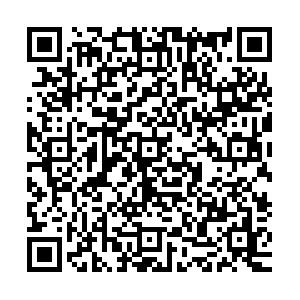# Analysis of the excited bottom and bottom-strange states B1(5721), ${{ B_{2}^{*}(5747) }}$, ${{ B_{s1}(5830)}}$, ${{B_{s2}^{*}(5840) }}$, ${{ B_{J}(5840) }}$and ${{B_{J}(5970) }}$of the B meson family• In order to make a further confirmation of the assignments of the excited bottom and bottom-strange mesons $B_{1}(5721)$, $B_{2}^{*}(5747)$, $B_{s1}(5830)$and $B_{s2}^{*}(5840)$and identify possible assignments of $B_{J}(5840)$and $B_{J}(5970)$, we study the strong decay of these states with the $^{3}P_{0}$decay model. Our analysis supports the assignments of $B_{1}(5721)$and $B_{2}^{*}(5747)$as the $1P_{1}'$and $1^{3}P_{2}$states, and $B_{s1}(5830)$and $B_{s2}^{*}(5840)$as the strange partners of $B_{1}(5721)$and $B_{2}^{*}(5747)$. Besides, we tentatively identify the recently observed $B_{J}(5840)$and $B_{J}(5970)$as the $2^{3}S_{1}$and $1^{3}D_{3}$states. It is noted that these conclusions need further confirmation by measurements of the decay channels $B_{J}(5840)\rightarrow B\pi$and $B_{J}(5970)\rightarrow B\pi$.
••Get Citation
Guo-Liang Yu and Zhi-Gang Wang. Analysis of the excited bottom and bottom-strange states B1(5721), ${{ B_{2}^{*}(5747) }}$, ${{ B_{s1}(5830)}}$, ${{B_{s2}^{*}(5840) }}$, ${{ B_{J}(5840) }}$and ${{B_{J}(5970) }}$of the B meson family[J]. Chinese Physics C, 2020, 44(3): 033103. doi: 10.1088/1674-1137/44/3/033103
Guo-Liang Yu and Zhi-Gang Wang. Analysis of the excited bottom and bottom-strange states B1(5721), ${{ B_{2}^{*}(5747) }}$, ${{ B_{s1}(5830)}}$, ${{B_{s2}^{*}(5840) }}$, ${{ B_{J}(5840) }}$and ${{B_{J}(5970) }}$of the B meson family[J]. Chinese Physics C, 2020, 44(3): 033103.Milestone
Article Metric

Article Views(356)
Cited by(0)
Policy on re-use
To reuse of Open Access content published by CPC, for content published under the terms of the Creative Commons Attribution 3.0 license (“CC CY”), the users don’t need to request permission to copy, distribute and display the final published version of the article and to create derivative works, subject to appropriate attribution.
###### 通讯作者: 陈斌, bchen63@163.com
• 1.

沈阳化工大学材料科学与工程学院 沈阳 110142

Title:
Email:

## Analysis of the excited bottom and bottom-strange states B1(5721), ${{ B_{2}^{*}(5747) }}$, ${{ B_{s1}(5830)}}$, ${{B_{s2}^{*}(5840) }}$, ${{ B_{J}(5840) }}$and ${{B_{J}(5970) }}$of the B meson family

• Department of Mathematics and Physics, North China Electric power university, Baoding 071003, China

Abstract: In order to make a further confirmation of the assignments of the excited bottom and bottom-strange mesons $B_{1}(5721)$, $B_{2}^{*}(5747)$, $B_{s1}(5830)$and $B_{s2}^{*}(5840)$and identify possible assignments of $B_{J}(5840)$and $B_{J}(5970)$, we study the strong decay of these states with the $^{3}P_{0}$decay model. Our analysis supports the assignments of $B_{1}(5721)$and $B_{2}^{*}(5747)$as the $1P_{1}'$and $1^{3}P_{2}$states, and $B_{s1}(5830)$and $B_{s2}^{*}(5840)$as the strange partners of $B_{1}(5721)$and $B_{2}^{*}(5747)$. Besides, we tentatively identify the recently observed $B_{J}(5840)$and $B_{J}(5970)$as the $2^{3}S_{1}$and $1^{3}D_{3}$states. It is noted that these conclusions need further confirmation by measurements of the decay channels $B_{J}(5840)\rightarrow B\pi$and $B_{J}(5970)\rightarrow B\pi$.

### HTML关注分享DownLoad:  Full-Size Img  PowerPoint